Open In App

# Turtle Programming in Python

“Turtle” is a Python feature like a drawing board, which lets us command a turtle to draw all over it! We can use functions like turtle.forward(…) and turtle.right(…) which can move the turtle around. Commonly used turtle methods are :

Plotting using Turtle

To make use of the turtle methods and functionalities, we need to import turtle.”turtle” comes packed with the standard Python package and need not be installed externally. The roadmap for executing a turtle program follows 4 steps:

1. Import the turtle module
2. Create a turtle to control.
3. Draw around using the turtle methods.
4. Run turtle.done().

So as stated above, before we can use turtle, we need to import it. We import it as :

```from turtle import *
# or
import turtle```

After importing the turtle library and making all the turtle functionalities available to us, we need to create a new drawing board(window) and a turtle. Let’s call the window as wn and the turtle as skk. So we code as:

```wn = turtle.Screen()
wn.bgcolor("light green")
wn.title("Turtle")
skk = turtle.Turtle()```

Now that we have created the window and the turtle, we need to move the turtle. To move forward 100 pixels in the direction skk is facing, we code:

`skk.forward(100)`

We have moved skk 100 pixels forward, Awesome! Now we complete the program with the done() function and We’re done!

`turtle.done()`

So, we have created a program that draws a line 100 pixels long. We can draw various shapes and fill different colors using turtle methods. There’s plethora of functions and programs to be coded using the turtle library in python. Let’s learn to draw some of the basic shapes.

Shape 1: Square

## Python

 `# Python program to draw square``# using Turtle Programming``import` `turtle``skk ``=` `turtle.Turtle()` `for` `i ``in` `range``(``4``):``    ``skk.forward(``50``)``    ``skk.right(``90``)``    ` `turtle.done()`

Output: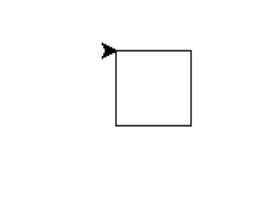Shape 2: Star

## Python3

 `# Python program to draw star``# using Turtle Programming``import` `turtle``star ``=` `turtle.Turtle()` `star.right(``75``)``star.forward(``100``)` `for` `i ``in` `range``(``4``):``    ``star.right(``144``)``    ``star.forward(``100``)``    ` `turtle.done()`

Output: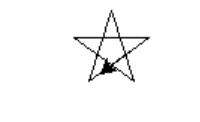Shape 3: Hexagon

## Python

 `# Python program to draw hexagon``# using Turtle Programming``import` `turtle``polygon ``=` `turtle.Turtle()` `num_sides ``=` `6``side_length ``=` `70``angle ``=` `360.0` `/` `num_sides` `for` `i ``in` `range``(num_sides):``    ``polygon.forward(side_length)``    ``polygon.right(angle)``    ` `turtle.done()`

Output: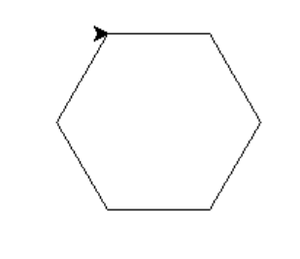## Python

 `import` `turtle` `# Initialize the turtle``t ``=` `turtle.Turtle()` `# Set the turtle's speed``t.speed(``1``)` `# Draw the parallelogram``for` `i ``in` `range``(``2``):``    ``t.forward(``100``)``    ``t.left(``60``)``    ``t.forward(``50``)``    ``t.left(``120``)`

Output: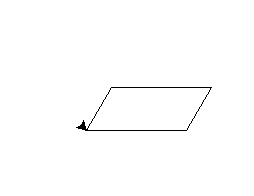## Python

 `import` `turtle` `# Set up the turtle screen and set the background color to white``screen ``=` `turtle.Screen()``screen.bgcolor(``"white"``)` `# Create a new turtle and set its speed to the fastest possible``pen ``=` `turtle.Turtle()``pen.speed(``0``)` `# Set the fill color to red``pen.fillcolor(``"red"``)``pen.begin_fill()` `# Draw the circle with a radius of 100 pixels``pen.circle(``100``)` `# End the fill and stop drawing``pen.end_fill()``pen.hideturtle()` `# Keep the turtle window open until it is manually closed``turtle.done()`

#### Output:Visit pythonturtle.org to get a taste of Turtle without having python pre-installed. The shell in PythonTurtle is a full Python shell, and you can do with it almost anything you can with a standard Python shell. You can make loops, define functions, create classes, etc.
You can access these codes for wonderful turtle programs here

### Some amazing Turtle Programs

1. Spiral Square Outside In and Inside Out

## Python

 `import` `turtle  ``#Inside_Out``wn ``=` `turtle.Screen()``wn.bgcolor(``"light green"``)``skk ``=` `turtle.Turtle()``skk.color(``"blue"``)` `def` `sqrfunc(size):``    ``for` `i ``in` `range``(``4``):``        ``skk.fd(size)``        ``skk.left(``90``)``        ``size ``=` `size ``+` `5` `sqrfunc(``6``)``sqrfunc(``26``)``sqrfunc(``46``)``sqrfunc(``66``)``sqrfunc(``86``)``sqrfunc(``106``)``sqrfunc(``126``)``sqrfunc(``146``)`

Output:

2. User Input Pattern

## Python

 `# Python program to user input pattern``# using Turtle Programming``import` `turtle   ``#Outside_In``import` `turtle``import` `time``import` `random` `print` `(``"This program draws shapes based on the number you enter in a uniform pattern."``)``num_str ``=` `input``(``"Enter the side number of the shape you want to draw: "``)``if` `num_str.isdigit():``    ``squares ``=` `int``(num_str)` `angle ``=` `180` `-` `180``*``(squares``-``2``)``/``squares` `turtle.up` `x ``=` `0``y ``=` `0``turtle.setpos(x, y)`  `numshapes ``=` `8``for` `x ``in` `range``(numshapes):``    ``turtle.color(random.random(), random.random(), random.random())``    ``x ``+``=` `5``    ``y ``+``=` `5``    ``turtle.forward(x)``    ``turtle.left(y)``    ``for` `i ``in` `range``(squares):``        ``turtle.begin_fill()``        ``turtle.down()``        ``turtle.forward(``40``)``        ``turtle.left(angle)``        ``turtle.forward(``40``)``        ``print` `(turtle.pos())``        ``turtle.up()``        ``turtle.end_fill()` `time.sleep(``11``)``turtle.bye()`

3. Spiral Helix Pattern

## Python

 `# Python program to draw``# Spiral  Helix Pattern``# using Turtle Programming` `import` `turtle``loadWindow ``=` `turtle.Screen()``turtle.speed(``2``)` `for` `i ``in` `range``(``100``):``    ``turtle.circle(``5``*``i)``    ``turtle.circle(``-``5``*``i)``    ``turtle.left(i)` `turtle.exitonclick()`

Output:

4. Rainbow Benzene

## Python

 `# Python program to draw``# Rainbow Benzene``# using Turtle Programming``import` `turtle``colors ``=` `[``'red'``, ``'purple'``, ``'blue'``, ``'green'``, ``'orange'``, ``'yellow'``]``t ``=` `turtle.Pen()``turtle.bgcolor(``'black'``)``for` `x ``in` `range``(``360``):``    ``t.pencolor(colors[x``%``6``])``    ``t.width(x``/``/``100` `+` `1``)``    ``t.forward(x)``    ``t.left(``59``)`

Output: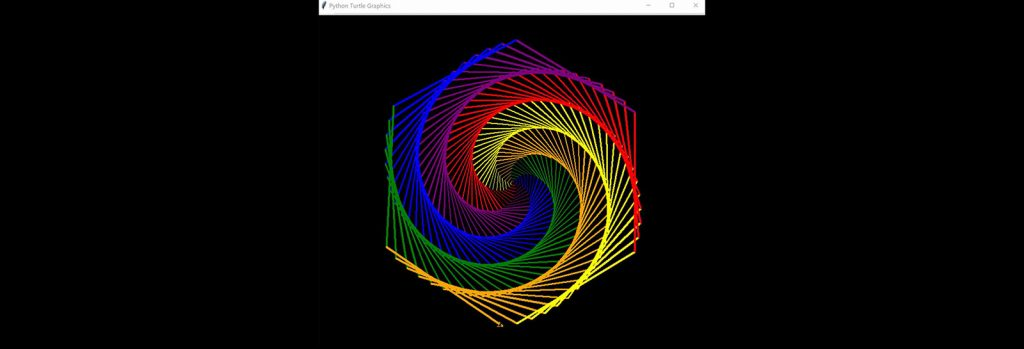Trees using Turtle Programming

References:

This article is contributed by Amartya Ranjan Saikia. If you like GeeksforGeeks and would like to contribute, you can also write an article using contribute.geeksforgeeks.org or mail your article to contribute@geeksforgeeks.org. See your article appearing on the GeeksforGeeks main page and help other Geeks.